# Chemical Engineering- CH 2017 GATE Paper MCQ Quiz

## 65 Questions MCQ Test GATE Past Year Papers for Practice (All Branches) | Chemical Engineering- CH 2017 GATE Paper MCQ Quiz

Description
Attempt Chemical Engineering- CH 2017 GATE Paper MCQ Quiz | 65 questions in 180 minutes | Mock test for GATE preparation | Free important questions MCQ to study GATE Past Year Papers for Practice (All Branches) for GATE Exam | Download free PDF with solutions
QUESTION: 1

### In a venturi meter, ∆P1 and ∆P2 are the pressure drops corresponding to volumetric flowrates Q1 and Q2. If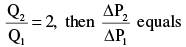Solution: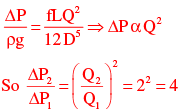QUESTION: 2

### The volumetric properties of two gases M and N are described by the generalized compressibility chart which expresses the compressibility factor (Z) as a function of reduced pressure and reduced temperature only. The operating pressure (P) and temperature (T) of two gases M and N along with their critical properties (PC , TC) are given in the table below.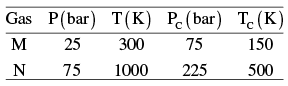ZM and ZN are the compressibility factor of the gases M and N under the given operating conditions respectively. The relation between ZM and ZN is

Solution: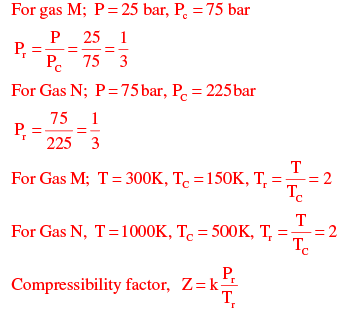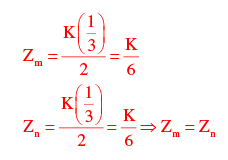QUESTION: 3

### Match the polymerization process in Group-1 with the polymers in Group-2.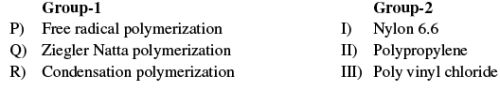Choose the correct set of combinations.

Solution: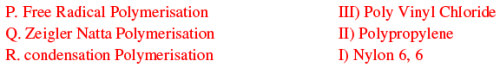QUESTION: 4

An LVDT (Linear Variable Differential Transformer) is a transducer used for converting

Solution:

LVDT converts displacement to voltage.

QUESTION: 5

Match the variables in Group-1 with the instruments in Group-2.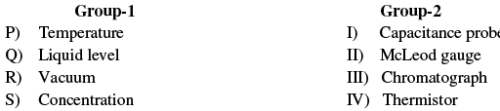Choose the correct set of combinations.

Solution:
QUESTION: 6

The thickness of laminar boundary layer over a flat plate varies along the distance from the leading edge of the plate. As the distance increases, the boundary layer thickness

Solution:
QUESTION: 7

The marks obtained by a set of students are 38, 84, 45,70, 75, 60, 48.  The mean and median marks, respectively, are

Solution: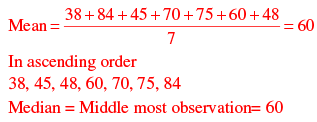*Answer can only contain numeric values
QUESTION: 8

In a heat exchanger, the inner diameter of a tube is 25 mm and its outer diameter is 30 mm. The overall heat transfer coefficient based on the inner area is 360 W/m2.°C. Then, the overall heat transfer coefficient based on the outer area, rounded to the nearest integer, is ___ W/m2.°C.

Solution: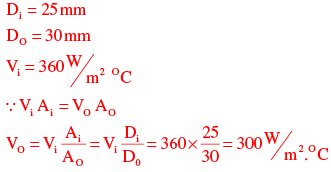*Answer can only contain numeric values
QUESTION: 9

The cost of a new pump (including installation) is 24,000 Rupees. The pump has useful life of 10 years. Its salvage value is 4000 Rupees. Assuming straight line depreciation, the book value of the pump at the end of 4th year, rounded to the nearest integer, is ___ Rupees.

Solution:

Cost of new pump =  Rs. 24000
Useful life of pump = 10 years
Salvage value of pump at the end of service life = 4000
Annual Depreciation using straight line method = (24000-4000)/10 = 2000 Rs
Book Value at the end of 4th year = Cost of equipment – Accumulated Depreciation till the end of 4th year
= 24000- (4*Annual depreciation)
= 24000-(4*2000)
=24000-8000
= 16000 Rs

*Answer can only contain numeric values
QUESTION: 10

A gas bubble (gas density ρg = 2 kg / m3; bubble diameter D = 10−4 m) is rising vertically through water (density ρ = 1000 kg / m3 ; viscosity µ = 0.001 Pa.s). Force balance on the bubble leads to the following equation,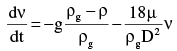Where ν is the velocity of the bubble at any given time t. Assume that the volume of the rising bubble does not change. The value of g = 9.81m / s2.  The terminal rising velocity of the bubble (in cm/s), rounded to 2 decimal places, is ___ cm/s.

Solution:

At terminal missing velocity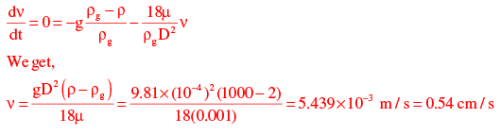QUESTION: 11

Water is heated at atmospheric pressure from 40°C to 80°C using two different processes. In process I, the heating is done by a source at 80°C. In process II, the water is first heated from 40°C to 60°C by a source at 60°C, and then from 60°C to 80°C by another source at 80°C. Identify the correct statement.

Solution:
QUESTION: 12

The DCDA (Double Contact Double Absorption) process is used the manufacture of

Solution:

The contact process is the current method of producing sulphuric acid in the high concentrations needed for industrial processes. Platinum used to be the catalyst for this reaction; however, as it is susceptible to reacting with arsenic impurities in the sulphuric feedstock, vanadium (V) oxide (V2O5) is now preferred.

*Answer can only contain numeric values
QUESTION: 13

The real part of 6eiπ/3 is ___.

Solution: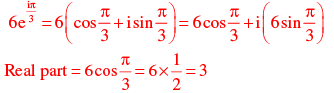QUESTION: 14

Consider a first order catalytic reaction in a porous catalyst pellet.
Given R - characteristic length of the pellet: De - effective diffusivity; kC - mass transfer coefficient: k1 - rate constant based on volume of the catalyst pellet; Cs - concentration of reactant on the pellet surface.

The expression for Thiele modulus is

Solution:
QUESTION: 15

Which of the following conditions are valid at the plait point?
P) Density difference between the extract and raffinate phases is zero
Q) Interfacial tension between the extract and raffinate phases is zero
R) Composition difference between the extract and raffinate phases is zero

Solution:

Plait point signifies the condition at which the composition of two liquid phases in equilibrium become identical and indeed transforms to a single phase. At this point interfacial tension and density difference between extract and raffinate phase is zero as both phases united to form one phase.

QUESTION: 16

The purpose of the mathanation reaction used in ammonia plants is to

Solution:
QUESTION: 17

Which of the following is the correct sequence of equipment for size reduction of solids?

Solution:

Solid is crushed into intermediate by jaw crusher after which grinding of solid is done by hammer mill. Ultrafine grinding is done by fluid energy mill.

QUESTION: 18

Let i and j be the unit vectors in the x and y directions, respectively. For the function F(x, y) = x3 + y3 the gradient of the function i.e.. ∇F is given by

Solution: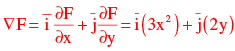QUESTION: 19

Consider steady state mass transfer of a solute A from a gas phase to a liquid phase. The gas phase bulk and interface mole fractions are yA,G and yA,i respectively. The liquid phase bulk and interface mole fractions are xA , L and xA ,i , respectively. The ratio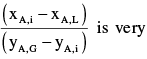close to zero.

The implies that mass transfer resistance is

Solution: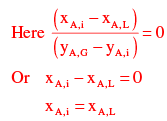Mole fraction of liquid phase interface is equal to mole fraction of liquid phase bulk.  It means resistance in the liquid phase is negligible.
Option (B) is correct

*Answer can only contain numeric values
QUESTION: 20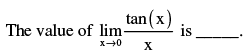Solution: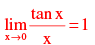QUESTION: 21

For a solid-catalyzed gas phase reversible reaction, which of the following statements is ALWAYS TRUE?

Solution:

Solid catalyst does not effect the equilibrium conversion.

QUESTION: 22

The one-dimensional unsteady heat conduction equation is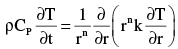Where, T – temperature, t – time, r – radial position, k – thermal conductivity, ρ- density, and
Cp - specific heat.

For the cylindrical coordinate system, the value of n in the above equation is

Solution:

n = 1 for cylindrical coordinate system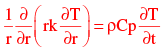*Answer can only contain numeric values
QUESTION: 23

The composition of vapour entering a tray in a distillation column is 0.47. The average composition of the vapour leaving the tray is 0.53. The equilibrium composition of the vapour corresponding to the liquid leaving this tray is 0.52. All the compositions are expressed in mole fraction of the more volatile component.

The Murphree efficiency based on the vapour phase, rounded to the nearest integer, is ___ %

Solution:

Murphree efficiency is defined as the actual change in average composition accomplished by a given tray divided by the change in average composition.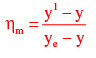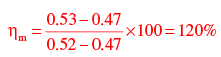Where,
y1 = Average composition of vapour leaving the tray.
ye = Equilibrium composition of vapour corresponding to the liquid leaving the tray.
y = Composition of vapour entering the tray.

*Answer can only contain numeric values
QUESTION: 24

The number of positive roots of the function f (x) shown below in the range 0 < x < 6 is  ___________.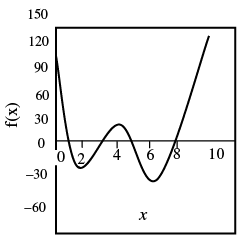Solution:

The graph of the function cuts x – axis in 3 times in 0 < x < 6
∴  The no. of positive roots=3

QUESTION: 25

The following reaction rate curve is shown for a reaction A → P. Here, (−rA) and xA represent reaction rate and conversion, respectively. The feed is pure A and 90% conversion is desired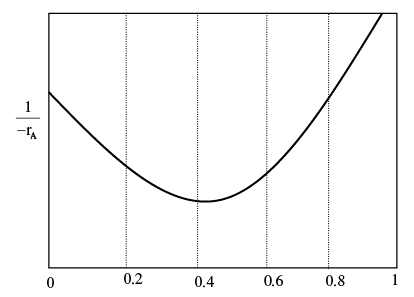Q. Which amongst the following reactor configurations gives the lowest total volume of the reactor (s)?

Solution:

The performance equation for a PFR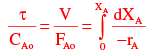Hence the volume required for the reactor for given conversion is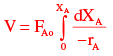Which is the area under curve between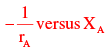The performance equation for a CSTR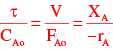Hence the volume required for the reactor for given conversion is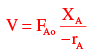Which is the volume of rectangle drawn on the graph between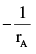versus XA for the given
conversion.
For the given reaction,
The following options give the following volumes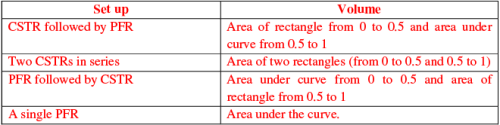Its clearly shown the combination of CSTR followed by PFR

*Answer can only contain numeric values
QUESTION: 26

The total cost (CT) of an equipment in terms of the operation variables x and y is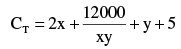The optimal value of CT, rounded to 1 decimal place, is ____.

Solution:

The total cost of an equipment in terms of the operating variables x and y is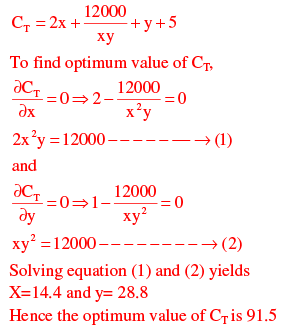*Answer can only contain numeric values
QUESTION: 27

The vapour pressure of a pure substance at a temperature T is 30bar. The actual and ideal gas values of g / RT for the saturated vapour at this temperature T and 30 bar are 7.0 and 7.7 respectively. Here, g is the molar Gibbs free energy and R is the universal gas constant.  The fugacity of the saturated liquid at these conditions, rounded to 1 decimal place, is ___ bar.

Solution: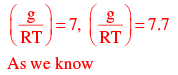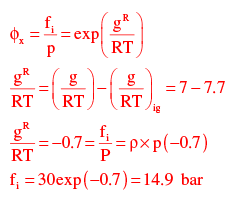QUESTION: 28

The Sherwood number (ShL) correlation for laminar flow over a flat plate of length L  is given by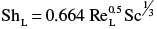Where ReL and Sc represent Reynolds number and Schmidt number, respectively.  This correlation, expressed in the form of Chilton-Colburn jD factor, is

Solution: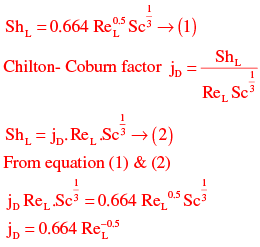*Answer can only contain numeric values
QUESTION: 29

For the initial value problem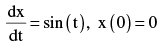the value of x at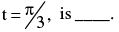Solution: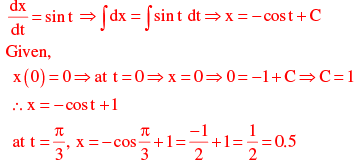*Answer can only contain numeric values
QUESTION: 30

In a counter current stripping operation using pure steam the mole ratio of a solute in the liquid stream is reduced from 0.25 to 0.05. The liquid feed flowrate, on a solute-free basis, is 3mole/s. The equilibrium line for the system is given in the figure below.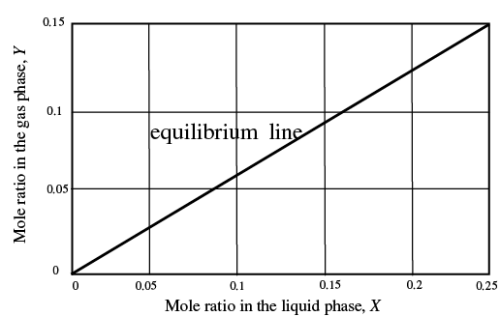The MINIMUM flowrate of pure steam for this process, rounded to 1 decimal place, is ___ mol/s.

Solution:

Equation of equilibrium line can be written as y = mX
m=slope of equilibrium line = 0.15/0.25 = 0.6
y = 0.6X
Material balance of solute gives L (0.25 − 0.05) = Gmin [0.6 (0.25)]
Here, L = 3mol / sec, G = minimum flow rate of pure steam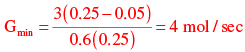QUESTION: 31

The Laplace transform of a function is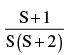. The initial and final values, respectively, of the function are

Solution: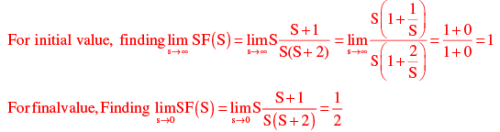QUESTION: 32

The following table provides four sets of Fanning friction factor data, for different values of Reynolds number (Re) and roughness factor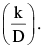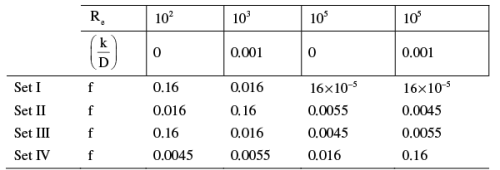Q. Which of the above sets of friction factor data is correct?

Solution:
*Answer can only contain numeric values
QUESTION: 33

In a batch adsorption process, 5 g of fresh adsorbent is used to treat 1 litre of an aqueous phenol solution. The initial phenol concentration is 100 mg/litre. The equilibrium relation is given by q* = 1.3C
Where, q* is the amount of phenol adsorbed in mg of phenol per gram of adsorbent: and C is the concentration of phenol in mg/litre in the aqueous solution.
When equilibrium is attained between the adsorbent and the solution, the concentration of phenol in the solution, rounded to 1 decimal place is ___________ mg/litre.

Solution:

Suppose y mg of phenol is adsorbed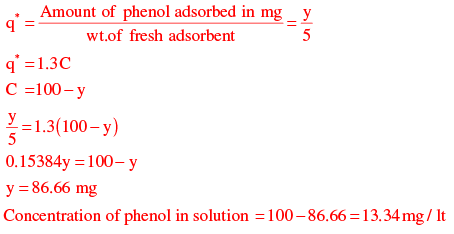*Answer can only contain numeric values
QUESTION: 34

A bond has a maturity value of 20,000 Rupees at the end of 4 years. The interest is compounded at the rate of 5% per year.

The initial investment to be made, rounded to the nearest integer, is _______ Rupees.

Solution:

Maturity value = 20000
Bond life = 4 years
Interest rate = 5%
Amount of investment to be made can be found from present worth formula  = 20000/(1+0.05)^4=16454.04=16454 Rs

*Answer can only contain numeric values
QUESTION: 35

Size analysis was carried out on a sample of gravel. The data for mass fraction (xi) and average particle diameter (Dpi) of the fraction is given in the table below: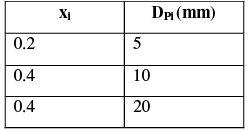The mass mean diameter of the sample, to the nearest integer, is ________mm.

Solution: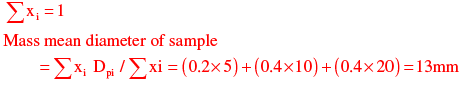*Answer can only contain numeric values
QUESTION: 36

A propeller (diameter D = 15 m) rotates at N = 1 revolution per second (rps). To understand the flow around the propeller, a lab-scale model is made. Important parameters to study the flow are velocity of the propeller tip (V = πND) , diameter D and acceleration due to gravity (g). The lab-scale model is 1/100th of the size of the actual propeller.

The rotation speed of the lab-scale model, to the nearest integer, should be _________ rps.

Solution:
*Answer can only contain numeric values
QUESTION: 37

The transfer function of a system is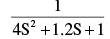For a unit step increase in the input, the fractional overshoot, rounded to 2 decimal places, is

Solution: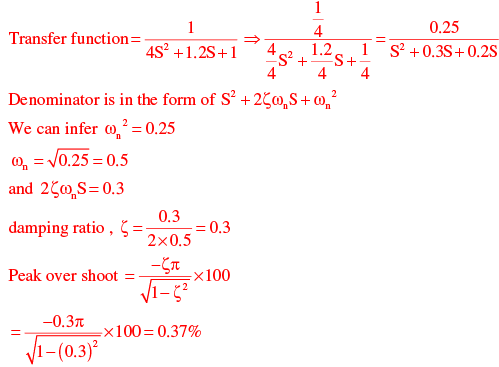QUESTION: 38

A fluid over a heated horizontal plate maintained at temperature Tw. The bulk temperature of the fluid is T. The temperature profile in the thermal boundary layer is given by: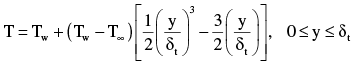Here, y is the vertical distance from the plate,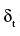is the thickness of the thermal boundary layer and k is the thermal conductivity of the fluid.

The local heat transfer coefficient is given by

Solution: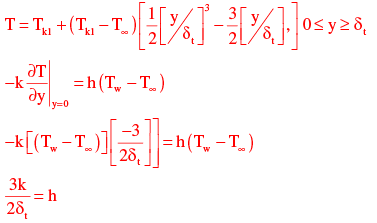*Answer can only contain numeric values
QUESTION: 39

The following liquid second-order reaction is carried out in an isothermal CSTR at steady state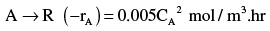Where, CA is the concentration of the reactant in the CSTR. The reactor volume is 2 m3 , the inlet flow rate is 0.5 m3 / hr and the inlet concentration of the reactant is 1000mol / m3 .

The fractional conversion, rounded to 2 decimal places, is _____.

Solution: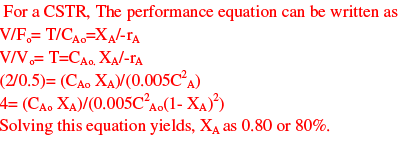*Answer can only contain numeric values
QUESTION: 40

Reaction A → B is carried out in a reactor operating at steady state and 1 mol/s of pure A at 425°C enters the reactor. The outlet stream leaves the reactor at 325°C. The heat input to the reactor is 17kW. The heat of reaction at the reference temperature of 25°C is 30kJ/mol. The specific heat capacities (in kJ/mol.K) of A and B are 0.1 and 0.15, respectively.

The molar flowrate of B leaving the reactor, rounded to 2 decimal places, is ____mol/s.

Solution:

A → B
Suppose X be the conversion of reactant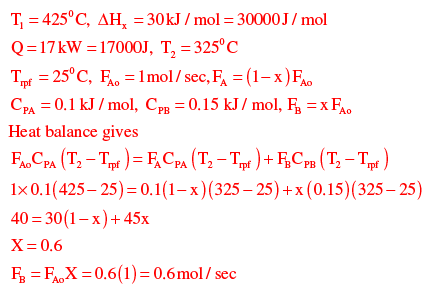*Answer can only contain numeric values
QUESTION: 41

The C-curve measured during a pulse tracer experiment is shown below. In the figure, C(t) is the concentration of the tracer measured at the reactor exit in mol/liter at time t seconds.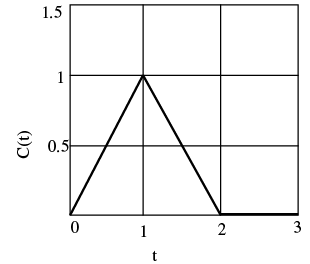The mean residence time in the reactor, rounded to 1 decimal place, is ______ s.

Solution:

The data can be drawn from the diagram is as follows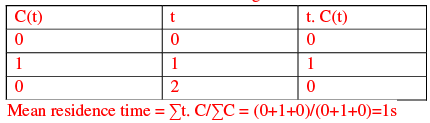*Answer can only contain numeric values
QUESTION: 42

The reversible reaction of t-butyl alcohol (TBA) and ethanol (EtOH) to ethylt-butyl ether (ETBE) is
TBA + EtOH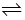ETBE + Water
The equilibrium constant for this reaction is KC = 1. Initially, 74 g of TBA is mixed with 100g of aqueous solution containing 46 weight% ethanol. The molecular weights are:74g/mol for TBA. 46g/mol for EtOH, 102g/mol for ETBE, and 18 g/mol for water.

The mass of ETBE at equilibrium, rounded to 1 decimal place, is ____g.

Solution:

Let x mole of ETBE present of equilibrium mass of EtOH=46 g
Mass of water = 54g

Moles of EtOH = 46/46mol  = 1 mol
Moles of water = 54/18 = 3 mol
TBA + EtOHETBE + water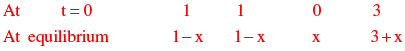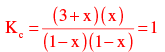x = 0.2
Moles of ETBE = 0.2
Moles of ETBE =  0.2 (102) = 20.4 gm

QUESTION: 43

Let Ibe the spectral black body radiation intensity per unit wavelength about the wavelength λ.

The blackbody radiation intensity emitted by a blackbody over all wavelengths is

Solution: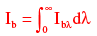QUESTION: 44

Match the equipment in Group-1 with the process in Group-2: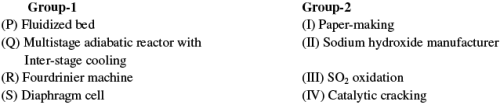Choose the correct set of combinations.

Solution:
*Answer can only contain numeric values
QUESTION: 45

Oil is being delivered at a steady state flowrate through a circular pipe of a radius 1.25 × 10 m−2
and length 10m. The pressure drop access the pipe is 500 Pa.

The shear stress at the pipe wall, rounded to 2 decimal places______ Pa

Solution:

r = 1.25 × 10 m−2m
L = 10 m, ∆P = 500 pa,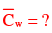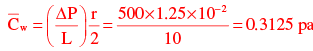QUESTION: 46

Match the problem type in Group-1 with the numerical method in Group-2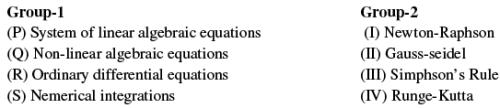Choose the correct set of combinations.

Solution:
*Answer can only contain numeric values
QUESTION: 47

The following gas-phase reaction is carried out in a constant-volume isothermal batch reactor A + B → R + S

The reactants A and B as well as the product S are non-condensable gases. At the operating temperature, the saturation pressure of the product R is 40 kPa.
Initially, the batch reactor contains equimolar amounts of A and B (and no products) at a total pressure of 100 kPa. The initial concentrations of the reactants are CA,0 = CB,0 = 12.56 mol / m3. The rate of reaction is given by (−rA) = 0.08CA CB mol / m3.s.

The time at which R just starts condensing, rounded to 1 decimal place, is_____

Solution:

A + B → R + S
Let m moles of A and B present initially in the reactor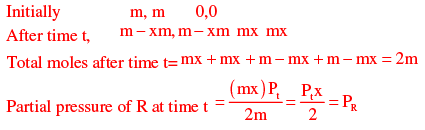At Pr =  40 kPa. R will start condensing
Here, Pt = 100kPa, PR = 40 = 100x\2
x = 0.8
CA =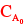(1 - X) = 1.25(1 - 0.8)
CA =  2.5mol / m3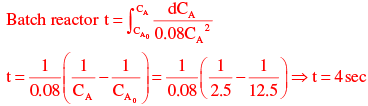*Answer can only contain numeric values
QUESTION: 48

The vapor phase composition and relative volatilities (with respect to n-propane) on an ideal tray of a distillation column are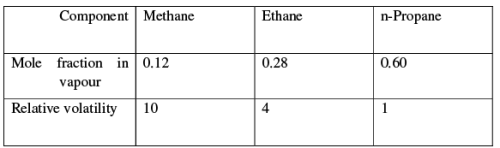The mole fraction of n-propane in the liquid phase, rounded to 2 decimal places, is ____.

Solution:

yA = 0.12, yB = 0.28, yC = 0.60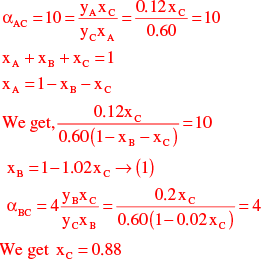*Answer can only contain numeric values
QUESTION: 49

The Characteristic equation of a closed-loop system is

6s3 + 11s2 + 6s + (1 + k) = 0, Where, k > 0

The value of K beyond which the system just becomes unstable, rounded to the nearest integer, is _____.

Solution:

Characteristic equation
6S3 + 11S2 + 6S + (1 + k) = 0
Use R-H criteria to find the value of k.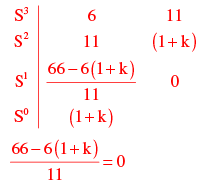66 - 6 (1 + k) = 0
66 = 6 (1 + k)
11 = 1 + k  k = 11 - 1 = 10
The value is 10.

*Answer can only contain numeric values
QUESTION: 50

A aqueous salt-solution enters a crystallizer operating at steady state at 25°C. The feed temperature is 900°C and the salt concentration in the feed is 40 weight%. The salt crystallizes as a pentahydrate. The crystals and the mother liquor leave the crystallizer. The molecular weight of the anhydrous salt is 135. The solubility of the salt at 25°C is 20 weight%.

The feed flowrate required for a production rate of 100 kg/s of hydrated salt, rounded to the nearest integer, is ____ kg/s.

Solution:

xF = Salt concentration in the feed (wt.%) = 0.4
Molecular wt. of 5H2 O molecules = 18 × 5 = 90
Molecular wt. of the anhydrous salt = 135
xC = wt% of hydrated crystal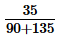= 0.6
xL = Solubility of salt = 0.2
Total Mass balance gives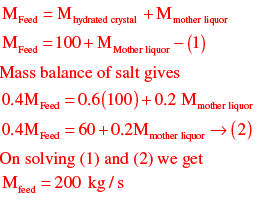*Answer can only contain numeric values
QUESTION: 51

The pressure of a liquid is increased isothermally. The molar volume of the liquid decreases from 50.45 × 10-6 m/ mol  to 48 × 10−6 m3 / mol during this process. The isothermal compressibility of the liquid is 10−9 Pa−1 , which can be assumed to be independent of pressure.

The change in the molar Gibbs free energy of the liquid, rounded to nearest integer, is ___ J/mol.

Solution: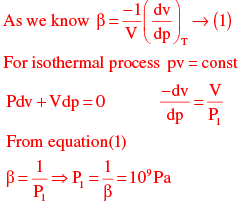At constant temperature
dG = Vdp - SdT ⇒ dG VdP = RT/P dP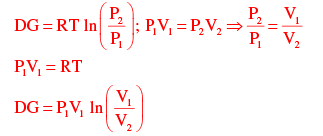P1 = 109 pa, V1 = 50.45×10-6 m3 / mol
V2 =  48×10-6 m3 / mol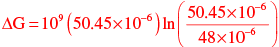ΔG = 50.45×103 In (1.051)
ΔG = 2511.48 J / mole

*Answer can only contain numeric values
QUESTION: 52

The open loop transfer function of a process with a proportional controller (gain KC) is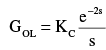Based on the Bode criterion for closed-loop stability, the ultimate gain of the controller, rounded to 2 decimal places, is____.

Solution: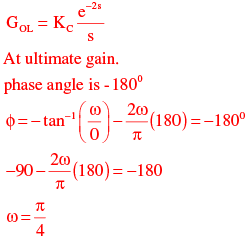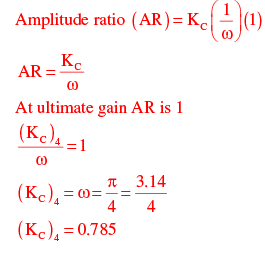QUESTION: 53

In nucleate boiling, the pressure inside a bubble is higher than the pressure of the surrounding liquid. Assuming that both the liquid and vapour are saturated, the temperature of the liquid will ALWAYS be

Solution:

The temperature of the liquid will always be lower than the temperature of the vapour

*Answer can only contain numeric values
QUESTION: 54

A box has 6 red balls and 4 white balls. A ball is picked at random and replaced in the box, after which a second ball is picked.  The probability of both the balls being red, rounded to 2 decimal places, is ____.

Solution: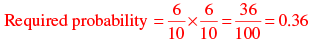*Answer can only contain numeric values
QUESTION: 55

A sparingly soluble gas (solute) is in equilibrium with a solvent at 10 bar. The mole fraction of the solvent in the gas phase is 0.01. At the operating temperature and pressure, the fugacity coefficient of the solute in the gas phase and the Henry’s law constant are 0.92 and 1000 bar, respectively. Assume that the liquid phase obeys Henry’s law.

The MOLE PERCENTAGE of the solute in the liquid phase, rounded to 2 decimal places, is _____.

Solution:

H = 1000 bar,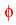= 0.92 for dilute solution
We can use Henry law for solute Hx =yP
(1000 x = 0.92 1 − 0.01) (10)
Here, y and x are mole fraction of solute in gas and liquid phase respectively
x = 9.108 ×10−3
Mole percentage of solute in liquid phase = 100 (9.108 × 10−3) = 0.91

QUESTION: 56

Consider the following sentences:
All benches are beds. No bed is a bulb. Some bulbs are lamps.
Which of the following can be inferred?
(i) Some beds are lamps.
(ii) Some lamps are beds.

Solution: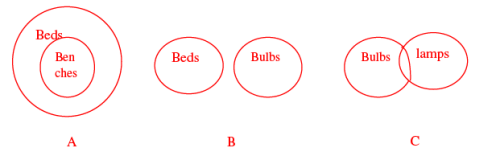Since, there is no direct relation given between lamps and beds. So, neither will be correct.

QUESTION: 57

The following sequence of numbers is arranged in increasing order: 1,x , x, x, y, y, 9,16,18.Given that the mean and median are equal, and are also equal to twice the mode, the value of y is

Solution: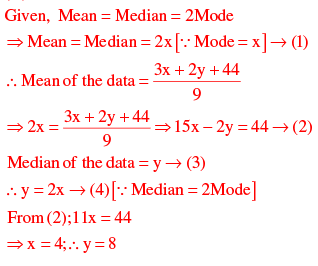QUESTION: 58

The bacteria in milk are destroyed when it _________ heated to 80 degree Celsius.

Solution:
QUESTION: 59

If the radius of a right circular cone is increased by 50%, its volume increases by

Solution:

Given, radius of a right circular cone is increased by 50%.
Let, height of the circular cone =(h)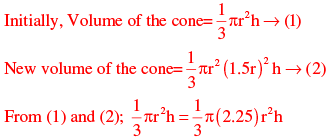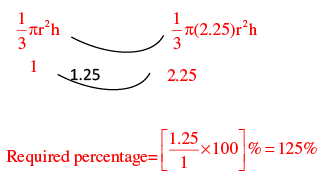QUESTION: 60

__________ with someone else’s email account is now very serious offence.

Solution:
QUESTION: 61

Students applying for hostel rooms are allotted rooms in order of seniority. Students already staying in a room will move if they get a room in their preferred list. Preferences of lower ranked applicants are ignored during allocation.

Given the data below, which room will Ajit stay in?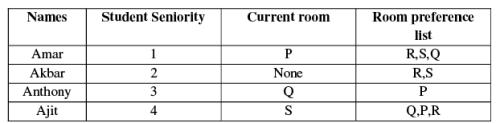Solution:

Amar → R
Akbar → S
Anthony → P
Ajit → Q
As per their preferences given.

QUESTION: 62

The bar graph below shows the output of five carpenters over one month, each of home made different items of furniture: Chairs, tables, and beds.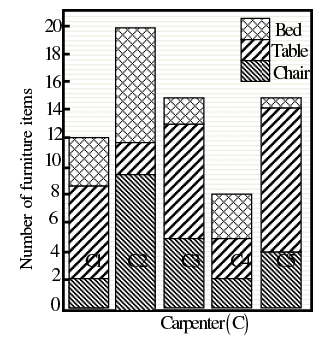Consider the following statements.
(i) The number of beds made by carpenter C2 is exactly the same as the same as the number  of tables made by carpenter C3.
(ii) The total number of chairs made by all carpenters is less than the total number of tables.

Q. Which one of the following is true?

Solution:

(i) The number of beds made by carpenter C2 is exactly the same as the number of tables made by carpenter C3
i.e., beds made by carpenter C2 = 8 = tables made by carpenter C3 [∵ From the bargraph]
So,(i) is correct.
(ii)  Total Number of tables made by all carpenters = 31.
Total Number of chairs made by all carpenters = 23
23 < 31
(ii) is correct

QUESTION: 63

The last digit of (2171)7 + (2172)9 + (2173)11 + (2174)13 is

Solution:

The last digit of (2171)7 = 1
The last digit of (2172)9 (2172)8 (2172)  = (2172)4n (2172) [Where, n = 2]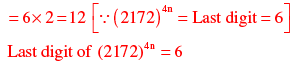The last digit of (2173)11 (2173)8 (2173)3
= (2173)4n (2173)3 [Where, n = 2]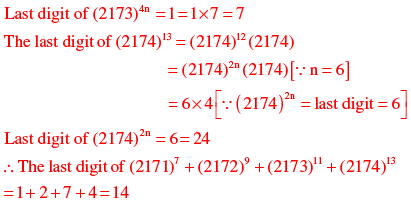QUESTION: 64

Two machines M1 and M2 are able to execute any of four jobs P, Q, R and S. The machines can perform one job on one object at a time. Jobs P, Q, R and S take 30 minutes, 20 minutes, 60 minutes and 15 minutes each respectively. There are 10 objects each requiring exactly 1 job. Job P is to be performed on 2 objects. Job Q on 3 objects. Job R on 1 object and Job S on 4 objects. What is the minimum time needed to complete all the jobs?

Solution: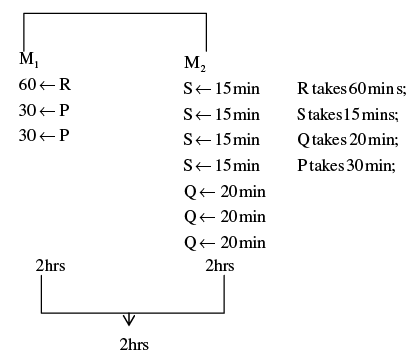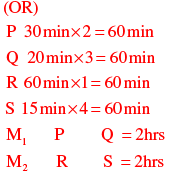QUESTION: 65

The old concert hall was demolished because of fears that the foundation would be affected by the construction tried to mitigate the impact of pressurized air pockets created by the excavation of large amounts of soil. But even with these safeguards, it was feared that the soil below the concert hall would not be stable.

From this, one can infer that

Solution:Use Code STAYHOME200 and get INR 200 additional OFF Use Coupon Code